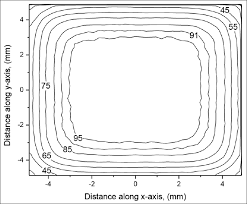# Draw Smooth option problem

hi
i want to draw a curve like this :i have rootfile and i a using this code for drawing :

``````TFile* f =new TFile("result.root");
TCanvas* c1 = new TCanvas("c1", "  ");
TTree* tree = (TTree*)f->Get("Ntuple1");
tree->Draw("x:y","pdd==10","C");
``````

but this is my output:

i would like to connect points to each other like first curve.

how can i do this?

thanks
result.root (605.1 KB)

Maybe @couet can give more details, but to draw contours only, use the `CONT1` or `CONT3` option

So a contour with any of the CONT option with not draw the plot you are looking for. For instance CONT1 gives:

if you use

``````tree->Draw("x:y","pdd==10","L");
``````

A graph with point line-connected will be produced but, has the points are not properly ordered, you will get:

The only way I can think of would be to extract the non empty bins from the histogram or the points of the TGraph when option L is used and order them properly to generate a contour.

1 Like

thank you all for your respondingi drawn all contour togather and used `CONT1` option and got this output

it is what i wanted.

is there a way to put number on each contour like first picture which i sent in first post ?
many thanks.

This is not foreseen by the option itself.
Somme example here: ROOT: tutorials/hist/ContourList.C File Reference